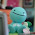## Thursday, January 13, 2011

### Final Percent Post

A percent means out of 100 and it also another name for hundreths
Ex. 57% means 57/100, 57 out of 100, or 0.57

4.1 Representing Percents
Representing percents means showing a percent as a picture in a hundred grid
Ex. 53% on a hundred grid, 53 squares of the grid would be shaded so its 53%

4.2 Fractions, Decimals, and Percents.
- Fractions, Decimals, and Percents can be used to represent numbers in various situations
- Percents can be written as a fraction and as decimal
Ex. 1/2% = 50%, 0.5% = 0.5/1000 = 0.005, 150% = 150/100 = 1.5, 42 3/4 = 42.75% = 42.75/100 = 0.4275

4.3 Percent of a number
- You can use mental math strategies such as halving, doubling, and dividing by ten to find the percent of same numbers
- To calculate the percent of a number, write the percent as a decimal then multiply by the numbers
Ex. 12 1/2% of 50, 0.125 x 50 = 6.25 (12 1/2% = 12.5% = 0.125)

4.4 Combining Percents
- Percent can be combined by adding to solve problems ; 5% + 7% = 12%
To calculate the increase in a number you can add the combined percent amount to the original number.

Ex. 12% of 100 = 0.12 x 100 = 12, 100 + 12 = 112
- You can multiply the original number by a single percent greater than 100
Ex. 112% of 100 = 1.12 x 100 = 112
- Percents of percents can be used to determine amounts that result from consecutive percent increases or decrease

Understanding Percents

My video.

1.